# DFT SYMMETRY

Looking at Figure 3-4(a) again, there is an obvious symmetry in the DFT results. Although the standard DFT is designed to accept complex input sequences, most physical DFT inputs (such as digitized values of some continuous signal) are referred to as real, that is, real inputs have nonzero real sample values, and the imaginary sample values are assumed to be zero. When the input sequence x(n) is real, as it will be for all of our examples, the complex DFT outputs for m = 1 to m = (N/2) – 1 are redundant with frequency output values for m > (N/2). The mth DFT output will have the same magnitude as the (N–m)th DFT output. The phase angle of the DFT's mth output is the negative of the phase angle of the (N–m)th DFT output. So the mth and (N–m)th outputs are related by the following: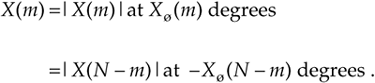We can state that when the DFT input sequence is real, X(m) is the complex conjugate of X(N–m), or

Equation 3-14'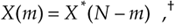where the superscript * symbol denotes conjugation.

In our example above, notice in Figures 3-4(b) and 3-4(d) that X(5), X(6), and X(7) are the complex conjugates of X(3), X(2), and X(1), respectively. Like the DFT's magnitude symmetry, the real part of X(m) has what is called even symmetry, as shown in Figure 3-4(c), while the DFT's imaginary part has odd symmetry, as shown in Figure 3-4(d). This relationship is what is meant when the DFT is called conjugate symmetric in the literature. It means that, if we perform an N-point DFT on a real input sequence, we'll get N separate complex DFT output terms, but only the first N/2+1 terms are independent. So to obtain the DFT of x(n), we need only compute the first N/2+1 values of X(m) where 0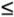m(N/2); the X(N/2+1) to X(N–1) DFT output terms provide no additional information about the spectrum of the real sequence x(n).

Although Eqs. (3-2) and (3-3) are equivalent, expressing the DFT in the exponential form of Eq. (3-2) has a terrific advantage over the form of Eq. (3-3). Not only does Eq. (3-2) save pen and paper, Eq. (3-2)'s exponentials are much easier to manipulate when we're trying to analyze DFT relationships. Using Eq. (3-2), products of terms become the addition of exponents and, with due respect to Euler, we don't have all those trigonometric relationships to memorize. Let's demonstrate this by proving Eq. (3-14) to show the symmetry of the DFT of real input sequences. Substituting N–m for m in Eq. (3-2), we get the expression for the (N–m)th component of the DFT:

Equation 3-15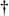Using our notation, the complex conjugate of x = a + jb is defined as x* = a – jb; that is, we merely change the sign of the imaginary part of x. In an equivalent form, if x = ejø, then x* = e–jø.

Because e–j2pn = cos(2pn) –jsin(2pn) = 1 for all integer values of n,

Equation 3-15'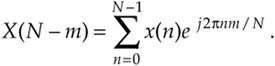We see that X(N–m) in Eq. (3-15') is merely X(m) in Eq. (3-2) with the sign reversed on X(m)'s exponent—and that's the definition of the complex conjugate. This is illustrated by the DFT output phase-angle plot in Figure 3-4(b) for our DFT Example 1. Try deriving Eq. (3-15') using the cosines and sines of Eq. (3-3), and you'll see why the exponential form of the DFT is so convenient for analytical purposes.

There's an additional symmetry property of the DFT that deserves mention at this point. In practice, we're occasionally required to determine the DFT of real input functions where the input index n is defined over both positive and negative values. If that real input function is even, then X(m) is always real and even; that is, if the real x(n) = x(–n), then, Xreal(m) is in general nonzero and Ximag(m) is zero. Conversely, if the real input function is odd, x(n) = –x(–n), then Xreal(m) is always zero and Ximag(m) is, in general, nonzero. This characteristic of input function symmetry is a property that the DFT shares with the continuous Fourier transform, and (don't worry) we'll cover specific examples of it later in Section 3.13 and in Chapter 5.

URL http://proquest.safaribooksonline.com/0131089897/ch03lev1sec2AmazonPrev don't be afraid of buying books Next